##Advanced Graphics Notes

Advanced Graphics, Dr Neil Dodgson, University of Cambridge Computer Laboratory
Part II course, 1999

• Overview
The course is divided into three sections:  I. 3D modelling techniques Dr Dodgson 6 lectures II. Human computer interaction Dr Blackwell 4 lectures III. Topics in graphics research Dr Dodgson 2 lectures
This handout covers section I. The other section's handouts will be given out in lectures. The purpose of the first section is to introduce you to a variety of 3D modelling and rendering techniques. The first lecture covers basic ideas, including a revision of the two standard methods of making images of 3D models: polygon scan conversion and ray tracing. The next two lectures delve into the depths of B-spline curves and surfaces, which are heavily used in many graphics applications. The fourth and fifth lectures consider four or five 3D modelling techniques. The final lecture introduces radiosity.
• Syllabus
The syllabus has ten parts, each maps to between a half and two lectures. This handout covers the first five parts.
1. Basic 3D modelling. Revision of the ray tracing, polygon scan conversion, and line drawing methods of making images from 3D models; the pros and cons of each approach. The polygon: drawing polygons; hardware speed-ups. Polygon mesh management: data structures.
2. Geometric primitives. Plane, polygon, sphere, cylinder, cone, box, disc, torus, quadrics, superquadrics. Ray intersection calculations for ray tracing. Converting the primitives into polygons for polygon scan conversion.
3. Splines. Revision of Bezier curves and surfaces. B-splines, from uniform, non-rational B-splines through to non-uniform, rational B-splines (NURBS).
4. Other 3D modelling mechanisms. Generative models: extrusion, revolution, sweeping, generalised cylinders. Constructive solid geometry (CSG): set theory applied to solid objects. Implicit surfaces and voxels: 3D pixels and the marching cubes algorithm.
5. Lighting. Revision of the basic diffuse + specular + ambient approximation. Radiosity: solving the inter-object diffuse reflection equations to produce more realistic images.
6. Introduction to HCI. Cycle of dialogue. The human virtual machine: Hick's Law, model human processor, Fitts' Law, power law of practice.
7. System evaluation. Usability analysis, GOMS, keystroke level modelling. Conceptual design and mental models.
8. User interface design. Cognitive walkthrough, design and evaluation of user trials.
9. Novel user interfaces.
10. Topics in graphics research. A taster of current research with detailed work in one or two areas selected from: free-form deformation, animation, natural object modelling.
• What's examinable?
Everything except where explicitely noted otherwise.
• Lecture handouts and supervision material
Some of the lecture course material is available on the web. This material is also printed out to provide these lecture notes. Other material is bound in with these notes (this material cannot be put on the web for copyright reasons). Point your supervisor at http://www.cl.cam.ac.uk/Teaching/1999/AGraphHCI. Supervisors please note that there are exercises scattered throughout the notes. These can usually be found at the end of sections.
• Book list and abbreviations
The following books are used in the course. Each is preceded by the abbreviation used on these pages to refer to that book.
FvDFH Foley, J.D., van Dam, A., Feiner, S.K. & Hughes, J.F. (1990). Computer Graphics: Principles and Practice. Addison-Wesley (2nd ed.).
F&vD Foley J.D. & van Dam, A. (1984). Fundamentals of Interactive Computer Graphics. Addison-Wesley (1st ed.).
R&A Rogers, D.F. & Adams, J.A. (1990). Mathematical Elements for Computer Graphics. McGraw-Hill (2nd ed.).
• Other abbreviations
RT ray tracing
PSC polygon scan conversion (e.g. z-buffer)
LD line drawing
SMAG Some Mathematics for Advanced Graphics, the mathematical supplement provided in the printed notes.
• Other material in the handout
In addition to these notes I have included copies of the following:
1. Lorenson and Cline's 1987 SIGGRAPH paper "Marching cubes: a high resolution 3D surface construction algorithm", Proc SIGGRAPH '87, pages 163�169. This is relevant to the section on voxels and that on marching cubes.
2. Pages 258-271 of Brian Wyvill's article "A Computer Animation Tutorial" in Computer Graphics Techniques: Theory and Practice, Rogers and Earnshaw (editors), Springer-Verlag, 1990, ISBN 0-387-97237-4. This is relevant to the section on implicit surfaces and also briefly mentions a use of super-ellipsoids on pages 264�265.
3. Extracts from Rogers and Adams (R&A): table 4-8 (conics), figure 6-18 (quadrics), and parts of sections 6-2 (surfaces of revolution), 6-3 (sweeps), 5-8 (Bezier curves), 5-9 (B-splines), and 5-13 (NURBS).
4. Some Mathematics for Advance Graphics (SMAG), my attempt to encapsulate the necessary mathematics for the work on ray tracing primitives (section 1), Bezier splines (section 2) and B-splines (sections 3 and 4).
5. The handwritten radiosity lecture notes.
The first three items in the above list are copyrighted material provided under the University of Cambridge's license from the Copyright Licensing Agency. This allows us to make one copy for each student and supervisor ("tutor") on the course within certain limits.These are: no more than three works and no more than 5% or one whole article or chapter from each work. This material is provided solely for the student's own study. Further copying of this handout is a breach of copyright.
Be warned: to fit inside these limits I have heavily edited the extracts from R&A. In particular, I have included none of the worked examples. To thoroughly understand the material I suggest that you read this extract and then buy or borrow a copy of R&A in order to go through the examples.
• Why HTML?
These notes are "typeset" in HTML. This is an experiment. HTML does not allow for particularly nice formatting in this printed copy of the notes. However, HTML does allow me to put a copy of the notes on the web, with full colour images (much better than the greyscale, half-toned images in this paper copy), and with lots of hyperlinks (especially in Part 1) for further investigation.
• Exercises
Scattered throughout these notes are exercises. My thanks to Jonathan Pfautz and Andy Penrose for some of the exercises.
 Exercises Exercises look like this.

# 1A) Ray tracing versus polygon scan conversion

These are the two standard methods of producing images of three-dimensional solid objects. They were covered in some detail in the Part IB course. If you want to revise them then check out FvDFH sections 14.4, 15.10 and 15.4 or F&vD sections 16.6 and 15.5. Line drawing is also used for representing three-dimensional objects in some applications. It is covered later on.

## Ray tracing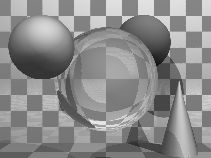Ray tracing has the tremendous advantage that it can produce realistic looking images. The technique allows a wide variety of lighting effects to be implemented. It also permits a range of primitive shapes which is limited only by the ability of the programmer to write an algorithm to intersect a ray with the shape.

Ray tracing works by firing one or more rays from the eye point through each pixel. The colour assigned to a ray is the colour of the first object that it hits, determined by the object's surface properties at the ray-object intersection point and the illumination at that point. The colour of a pixel is some average of the colours of all the rays fired through it. The power of ray tracing lies in the fact that secondary rays are fired from the ray-object intersection point to determine its exact illumination (and hence colour). This spawning of secondary rays allows reflection, refraction, and shadowing to be handled with ease.

Ray tracing's big disadvantage is that it is slow. It takes minutes, or hours, to render a reasonably detailed scene. Until recently, ray tracing had never been implemented in hardware. A Cambridge company, Advanced Rendering Technologies, is trying to do just that, but they will probably still not get ray tracing speeds up to those achievable with polygon scan conversion.

Ray tracing is used where realism is vital. Example application areas are high quality architectural visualisations, and movie or television special effects.

### Example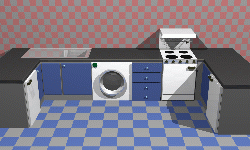This kitchen was rendered using the ray tracing program rayshade.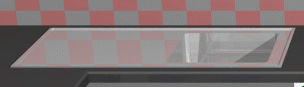These close-ups of the kitchen scene show some of the power of ray tracing. The kitchen sink reflects the wall tiles. The benchtop in front of the kitchen sink has a specular highlight on its curved front edge.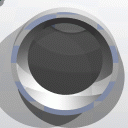The washing machine door is a perfectly curved object (impossible to achieve with polygons). The inner curve is part of a cone, the outer curve is a a cylinder. You can see the floor tiles reflected in the door. Both the washing machine door and the sink basin were made using CSG techniques (see Part 4C).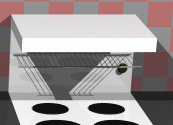The grill on the stove casts interesting shadows (there are two lights in the scene). This sort of thing is much easier to do with ray tracing than with polygon scan conversion.

## Polygon scan conversionThis term encompasses a range of algorithms where polygons are rendered, normally one at a time, into a frame buffer. The term scan comes from the fact that an image on a CRT is made up of scan lines. Examples of polygon scan conversion algorithms are the painter's algorithm, the z-buffer, and the A-buffer (FvDFH chapter 15 or F&vD chapter 15). In this course we will generally assume that polygon scan conversion (PSC) refers to the z-buffer algorithm or one of its derivatives.

The advantage of polygon scan conversion is that it is fast. Polygon scan conversion algorithms are used in computer games, flight simulators, and other applications where interactivity is important. To give a human the illusion that they are interacting with a 3D model in real time, you need to present the human with animation running at 10 frames per second or faster. Polygon scan conversion can do this. The fastest hardware implementations of PSC algorithms can now process millions of polygons per second.

One problem with polygon scan conversion is that it can only support simplistic lighting models, so images do not necessarily look realistic. For example: transparency can be supported, but refraction requires the use of an advanced and time-consuming technique called "refraction mapping"; reflections can be supported -- at the expense of duplicating all of the polygons on the "other side" of the reflecting surface; shadows can be produced, by a more complicated method than ray tracing. The other limitation is that it only has a single primitive: the polygon, which means that everything is made up of flat surfaces. This is especially unrealistic when modelling natural objects such as humans or animals. An image generated using a polygon scan conversion algorithm, even one which makes heavy use of texture mapping, will tend to look computer generated.

### Examples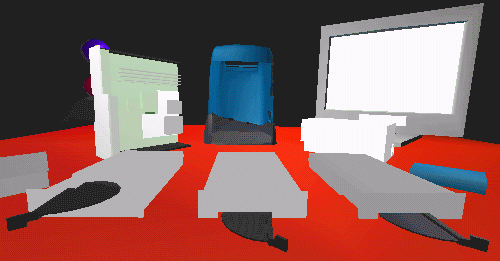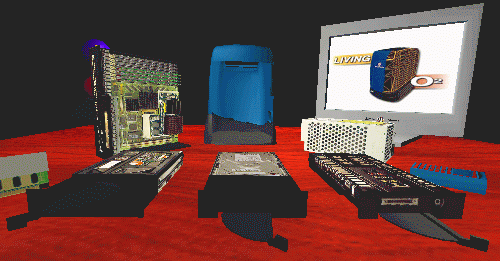Texture mapping is a simple way of making a PSC (or a RT) scene look better without introducing lots of polygons. The image above left shows a scene without any texture maps. The equivalent scene with texture maps is shown above right. Obviously this scene was designed to be viewed with the texture maps turned on. This example shows that texture mapping can make very simple geometry look interesting to a human observer.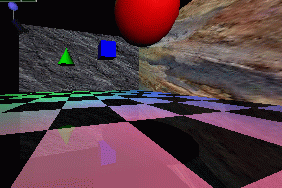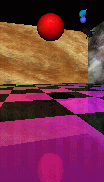The image at left was generated using PSC. Texture mapping has been used to make the back and side walls more interesting. All the objects are reflected in the floor. This reflection is achieved by duplicating all of the geometry, upside-down, under the floor, and making the floor partially transparent. The close-up at right shows the reflection of the red ball, along with a circular "shadow" of the ball. This shadow is, in fact, a polygonal approximation to a circle drawn on the floor polygon and bears no relationship to the lights whatsoever.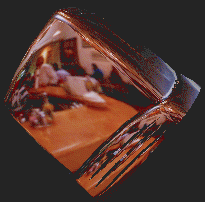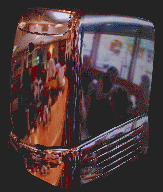Environment mapping is another clever idea which makes PSC images look more realistic. In environment mapping we have a texture map of the environment which can be thought of as wrapping completely around the entire scene (you could think of it as six textures on the six inside faces of a big box). The environment map itself is not drawn, but if any polygon is reflective then the normal to the polygon is found at each pixel (this normal is needed for Gouraud shading anyway) and from this the appropriate point (and therefore colour) on the environment map can be located. This example shows a silvered SGI O2 computer reflecting an environment map of the interior of a cafe.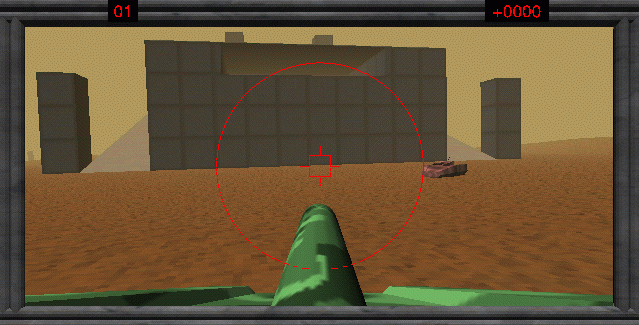PSC is, of course, widely used in interactive games. Here we see an incautious opponent about to drive into our player's sights. The graphics are not particularly sophisticated: there are very few polygons in the scene, but the scene is made more interesting, visually, by using texture mapping. When playing the game people tend to worry more about winning (or, in some cases, not losing too badly) than about the quality of the graphics. Graphical quality is arguably more useful in selling the game to the player than in actual game play.

## Line drawing

An alternative to the above methods is to draw the 3D model as a wire frame outline. This is obviously unrealistic, but is useful in particular applications. The wire frame outline can be either see through or hidden lines can be removed (FvDFH section 15.3 or F&vD section 14.2.6). In general, the lines that are drawn will be the edges of the polygons which would be drawn by a PSC algorithm.

Line drawing is generally faster than PSC unless the PSC is being done by specialist hardware. Line drawing of 3D models is used in Computer Aided Design (CAD) and in 3D model design. The software which people use to design 3D models for ray tracing tends to use both LD and PSC in its user interface. It is interesting to note that, when R&A was first written (1976), the authors had only line drawing algorithms with which to illustrate their 3D models. The only figure in the entire book which does not use exclusively line drawing is Fig. 6-52, which has screen shots of a prototype PSC system.

## Out in the real world...

3D graphics finds applications in three main areas:
• visualisation -- scientific, medical, and architectural
• simulation -- for example, flight simulators
• entertainment -- movie and TV special effects and games

Visualisation generally does not require realistic looking images. In science we are usually visualising complex three dimensional structures, such as protein molecules, which have no "realistic" visual analogue. In medicine we generally prefer an image that helps in diagnosis over one which looks beautiful. PSC is therefore normally used in visualisation (although some data require voxel rendering -- see Part 4D).

Simulation uses PSC because it can generate images at interactive speeds. At the high (and very expensive) end a great deal of computer power is used. In the most expensive flight simulators (those with full hydraulic suspension and other fancy stuff) the graphics kit can cost �1,000,000 out of a total cost of about ten times that figure.

3D games (for example Quake, Unreal, Descent) use PSC because it gives interactive speeds. A lot of other "3D" games (for example SimCity, Civilisation, Diablo) use pre-drawn sprites (small images) which they simply copy to the appropriate position on the screen. This essentially reduces the problem to an image compositing operation, requiring much less processor time. The sprites can be hand drawn by an artist or created in a 3D modelling package and rendered to sprites in the company's design office. Donkey Kong Country, for example, uses sprites which were ray traced from 3D models.

You may have noticed that the previous sentence is the first mention of ray tracing in this section. It transpires that the principal use of ray tracing, in the commercial world, is in producing special effects for movies and television.

The first movie to use 3D computer graphics was Star Wars . You may remember that there were some line drawn computer graphics toward the end of the movie. All of the spaceship shots, and all of the other fancy effects, were done using models, mattes (hand-painted backdrops), and hand-painting on the actual film. Computer graphics technology has progressed incredibly since then. The recent re-release of the Star Wars trilogy included a number of computer graphic enhancements, all of which were composited into the original movie.

A recent example of computer graphics in a movie is the (rather bloodythirsty) Starship Troopers . Most of the giant insects in the movie are completely computer generated. The spaceships are a combination of computer graphic models and real models. The largest of these real models was 18' (6m) long: so it is obviously still worthwhile spending a lot of time and enrgy on the real thing.

Special effects are not necessarily computer generated. Compare King Kong  with Godzilla . The plots have not changed that much, but the special effects have improved enormously: changing from hand animation (and a man in a monkey suit) to swish computer generated imagery. Not every special effect you see in a modern movie is computer generated. In Starship Troopers, for example, the explosions are real. They were set off by a pyrotechnics expert against a dark background (probably the night sky), filmed, and later composited into the movie. In Titanic  the scenes with actors in the water were shot in the warm Gulf of Mexico. In order that they look as if they were shot in the freezing North Atlantic, cold breaths had to be composited in later. These were filmed in a cold room over the course of one day by a special effects studio.Film makers obviously need to balance quality, ease of production, and cost. They will use whatever technology gives them the best trade off. This is increasingly computer graphics, but computer graphics is still not useful for everything by quite a long way.

A recent development is the completely computer generated movie. Toy Story  was the world's first feature length computer generated movie. Two more were released last year (A Bug's Life  and Antz ). Toy Story 2  will be released in the UK on 4 February 2000.

## PSC or RT for SFX?

While RT gives better results than PSC, we can often get acceptable results with PSC through the use of techniques such as environment mapping and the use of lots and lots and lots of tiny polygons. The special effects industry still dithers over whether to jump in and use RT. Many special effects are done using PSC, with maybe a bit of RT for special things (giving a hybrid RT/PSC algorithm). Toy Story, for example, used Pixar's proprietary PSC algorithm. It still took between 1 and 3 hours to render each frame (although you must remember that these frames have a resolution of about 2,000 by 1,000 pixels). More expensive algorithms can be used in less time if you are rendering for television (I estimate that about one sixth of the pixels are needed compared to a movie) or if you are only rendering a small part of a big image for compositing into live action.

Finally, at SIGGRAPH 98 I had the chance to hear about the software that some real special effects companies are using:

BlueSky|ViFX
Everything is ray traced using CGI-Studio.
Digital Domain
Use ray tracing in commercial software, except when the commercial software cannot do what they want. Used MentalRay on Fifth Element ; used Alias models (NURBS) passed to Lightwave (polygons) for one advertisement; used MentalRay plus Renderman for another advertisement.
Rhythm + Hues
Use a proprietry renderer, which is about 10 years old. It has been rewritten many times. They make only limited use of ray tracing.
Station X
Use Lightwave plus an internally developed renderer which is a hybrid between ray tracing and z-buffer.
 Exercises Compare and contrast the capabilities and uses of ray tracing and polygon scan conversion. In what circumstances is line drawing more useful than either ray tracing or polygon scan conversion. (a) When is realism critical? Give 5 examples of applications where different levels of visual realism are necessary. Choose ray tracing or polygon scan conversion for each application and explain why you made your choice. (b) How would you determine what level of visual realism is `necessary' for a given application? "The quality of the special effects cannot compensate for a bad script." Discuss with reference to (recent) movies that you have seen.

# 1B) Polygon mesh management

Relevant mainly to PSC and LD.

## Drawing polygons

In order to draw a polygon, you obviously need to know its vertices. To get the shading correct you also need to know its orientation: which side is the front of the polygon and which is the back. Many systems assume one-sided polygons: the front side is shaded and the back side either is coloured matt grey or black or is not even considered. This is sensible if the polygon is part of a closed polyhedron. In many applications, all objects consist of closed polyhedra; but you cannot guarantee that this will always be the case, which means that you will get unexpected results when the back sides of polygons are actually visible on screen.

The orientation of a polygon is specified by its normal vector. This does not need to be specified independently of the polygon's vertices because it can be calculated from the vertices. As an example: assume a polygon has three vertices, A, B and C. The normal vector can be calculated as: n = (C-B) x (A-B).

Any three adjacent vertices in a polygon can be used to calculate the normal vector but the order in which the vertices are specified is important: it changes whether the vector points up or down relative to the polygon. In a right-handed co-ordinate system the three vertices must be specified anti-clockwise round the polygon as you look down the desired normal vector (i.e. as you look at the front side of the polygon). Note that, if there are more than three vertices in the polygon, they must all lie in the same plane, otherwise the shape will not be a polygon!

Thus, for drawing purposes, we need to know only the vertices and surface properties of the polygon. The vertices naturally give us both edge and orientation information. The surface properties are such things as the specular and diffuse colours, and details of any texture mapping which may be applied to the polygon.

## Interaction with polygon mesh data

The above is fine for drawing but, if you wish to manipulate the polygon mesh (for example, in a 3D modelling package), then is is useful to know quite a lot more about the connectivity of the mesh. For example: if you want to move a vertex, which is shared by four polygons, you do not want to have to search through all the polygons in your data structure trying to find the ones which contain a vertex which matches your vertex data.

The winged-edge data structure is particularly useful for handling polygon mesh data. It contains explicit links for all of the relationships between vertices, edges and polygons, thus making it easy to find, for example, which polygons are attached to a given vertex, or which polygons are adjacent to a given polygon (by traversing the edge list for the given polygon, and finding which polygon lies on the other side of each edge).

The vertex object contains the vertex's co-ordinates, a pointer to a list of all edges of which this vertex is an end-point, and a pointer to a list of all polygons of which the vertex is a vertex.

The polygon object contains (a pointer to) the polygon's surface property information, a pointer to a list of all edges which bound this polygon, and a pointer to an ordered list of all vertices of the polygon.

The edge object contains pointers to its start and end vertices, and pointers to the polygons which lie to the left and right of it.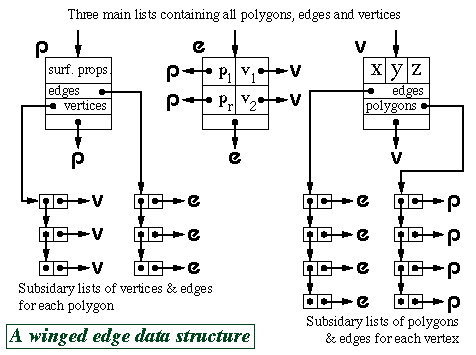The above diagram shows just one possible implementation of a polygon mesh data structure. FvDFH section 12.5.2 describes another winged-edge data structure which contains slightly less information, and therefore requires more accesses to find certain pieces of information than the one shown above. The implementation that would be chosen depends on the needs of the particular application which is using the data structure. Another alternative would be to attach shading information to vertices rather than to polygons. This could then be used to Gouraud shade or Phong shade the polygons.

F&vD section 13.2 also contains a bit of information on polygon meshes.

# Hardware PSC quirks

A piece of PSC hardware, such as the Silicon Graphics Reality Engine, will generally consist of a geometry engine and a rendering engine. The geometry engine will handle the transformations of all vertices and normals (and possibly handle some of the shading calculations). The rendering engine will implement the PSC algorithm on the transformed data.

## Triangles only

When making a piece of hardware to render a polygon, it is much easier to make the hardware handle a fixed number of vertices per polygon, than a variable number. Many hardware implementations thus simply implement triangle drawing. This is not a serious drawback. Polygons with more vertices are simply split into triangles.

## The triangle strip set and triangle fan set

In addition to simple triangle drawing, Silicon Graphics machines implement both the triangle strip set and triangle fan set to speed up processing through the geometry engine. Each triangle in the set has two vertices in common with the previous triangle. Each vertex is transformed only once by the geometry engine, giving a factor of three speed up in geometry processing.

For example, assume we have triangles ABC, BCD, CDE and DEF. In standard triangle rendering, the vertices would be sent to the geometry engine in the order ABC BCD CDE DEF; each triangle's vertices being sent separately. With a triangle strip set the vertices are sent as ABCDEF; the adjacent triangles' vertices overlapping.

A triangle fan set is similar. In the four triangle case we would have triangles ABC, ACD, ADE and AEF. The vertices would again be sent just as ABCDEF. It is obviously important that the rastering engine be told whether it is drawing standard triangles or a triangle strip set or a triangle fan set.

 Exercises Calculate both surface normal vectors (left-handed and right-handed) for a triangle with points (1, 1, 0), (2, 0, 1), (-1, -2, -1). Confirm that the following statements are correct for a reasonable polygon mesh: A vertex belongs to at least two edges. A vertex is a vertex of at least one polygon. An edge has exactly two end points. An edge is an edge of either one or two polygons. A polygon has at least three vertices. A polygon has at least three edges. Work out the algorithm that is required to modify a winged-edge data structure when an edge is split. You may ignore surface property information for the polygons and you may assume that the edge that is split is split exactly in half. The algorithm could by called by the function call: split_edge( vertex_list v, edge_list e, polygon_list p, edge edge_to_split) where the winged-edge data structure is made up of the three linked lists of objects (vertices, edges, and polygons).

# 2A) Ray tracing primitives

Relevant mainly to RT.

A primitive is a shape for which a ray-shape intersection routine has been written. More complex objects can be built out of the primitives. Most ray tracers will have a variety of primitives. They are limited only by the ability of the programmer to write a function to analytically intersect a ray with the surface of the shape.

## Common primitives

Plane
The infinite plane is a simple object with which to intersect a ray (see Part IB notes for details). On its own it can represent boundary objects such as the ground or the sky or perhaps an infinite wall. Intersection with the infinite plane is a useful building block in a ray tracing system.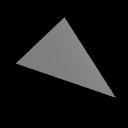Polygon
Having the polygon as a ray tracing primitive allows a ray tracer to render anything that a PSC algorithm could. To find the intersection of a ray with a polygon, first find the intersection of the ray with the infinite plane in which the polygon lies. Then ascertain whether the intersection lies inside or outside the polygon: this is a reasonably straightforward two dimensional graphics operation.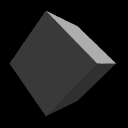Box
A box is essentially six polygons and could be ray traced as such. However, intersection with an axis-aligned box can be optimised. Any box can be axis-aligned by appropriate transformations. We can thus write a routine to intersect an arbitrary ray with an axis-aligned box and then transform the ray under consideration in exactly the same way as we transform the box which we are trying to intersect with it. This sort of idea generalises neatly to the concept of specifying any object in a convenient object coordinate system and then applying transforms to the whole object to place it at the appropriate point in the world coordinate system.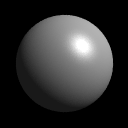Sphere
The sphere is the simplest finite object with which to intersect a ray. Practically any ray tracing program will include the sphere as a primitive. Scaling a sphere by different amounts along the different axes will produce an ellipsoid: a squashed or stretched sphere. There is thus no need to include the ellipsoid as a primitive provided that your ray tracer contains the usual complement of transformations. (It would be a poor ray tracer if it did not!)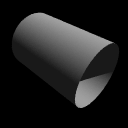Cylinder
Intersecting a ray with an infinitely long cylinder is practically as easy as intersecting one with a sphere. The tricky bit, if it can be called that, is to intersect a ray with a more useful finite length cylinder. This is achieved by intersecting the ray with the appropriate infinitely long cylinder and then ascertaining where along the cylinder the intersection lies. If it lies in the finite length in which you are interested then keep the intersection. If it does not then ignore the intersection. Note that the ray tracer used to render the accompanying image has cylinders without end caps. This is the correct result if you follow the procedure outlined in this paragraph. Adding end caps to your cylinders requires extra calculations.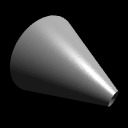Cone
Cones are very like cylinders. Like the infinite cylinder, there is a simple mathematical definition of an infinite cone which makes it easy to write a ray-cone intersection algorithm. Note that a cone does not need to have a point -- it can be truncated short of its `top', as illustrated in the accompanying image. The particular ray tracer used does not add end caps to cones.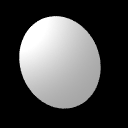Disc
The disc is not a common ray tracing primitive, but is necessary in ray tracers which implement cones and cylinders without end caps. It is handled in much the same way as the polygon. The routine to check if the intersection with the plane lies inside or outside of the disc is simpler than the equivalent routine for the polygon. In the accompanying ray traced image, the disc has been positioned so that it just catches the light -- this illustrates how specular reflection varies across a completely flat surface. The sphere, cone and torus images illustrate how specular reflection varies across three curved surfaces.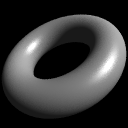Torus
Toroids are reasonably rare in real life (doughnuts and tyre inner tubes notwithstanding). They are somehow alluring to the kinds of people who implement ray tracers and, having a reasonably straightforward mathematical definition, are reasonably simple to implement. They thus appear as primitives in many ray tracers. They become more useful when combined with Constructive Solid Geometry (see Part 4C).

## Ray-primitive intersections

SMAG section 1 contains the mathematics of ray-primitive intersections and details of how you would go about converting a primitive object into polygons for PSC.

 Exercises Work out mathematical equations to define a plane, a sphere, an infinitely long cylinder, an infinitely long cone, and a torus. You will find it helpful to centre each primitive at the origin and to align it in a sensible way with respect to the coordinate axes. You may like to use cylindrical polar coordinates where these prove useful. As a starting point remember that a circle can be represented by the equation: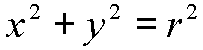Work out a way to intersect a ray with each of the five primitives. You may assume that you are provided with functions to find the roots of linear, quadratic, cubic and quartic equations. Work out if there exists a faster intersection algorithm for an axis aligned 2x2x2 unit box than just six separate polygon intersection calculations (N.B. I don't know whether there is or not). [1999/7/11] (a) Give a parametric definition of a torus centred at the origin and aligned with the coordinate axes. (b) Outline how you would find the first intersection point, if any, of a ray with the torus from the previous part. Show how to convert a cylinder into a polygon mesh. What changes do you have to make if the mesh may contain only triangles? Show how to convert a torus into a polygon mesh. Show how to convert a sphere into a triangle mesh. How can you get the most even distributiuon of triangle vertices across the sphere?

The ray tracing primitives, described in Part 2A, have relatively simple mathematical definitions. This is what makes them attractive: the simple mathematical definition allows for simple ray-object intersection code. Following from this, it would seem logical to investigate other shapes with simple mathematical definitions. Spheres, cones and cylinders are part of a more general family of parametric surfaces called quadrics (N.B. tori are not quadrics). Quadrics are the 3D analogue of 2D conics. We describe these general families below, but it turns out that they are of little practical use. It would seem that the general quadrics are a "dead end" in graphics research.

## Conics

A conic is a two dimensional curve desribed by the general equation: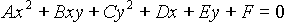This general form can be rotated, scaled, and translated so that it is aligned along the axes of the coordinate system. It will then have the simpler equation: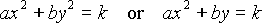The useful conics are the ellipse (of which the circle is a special case), the hyperbola, and the parabola. For more details see R&A section 4-10, especially Table 4-8 on page 242. Table 4-8 is included in the handout.

The quadrics are the three dimensional analogue of the conics. The general equation is: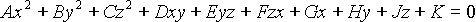This general form can be rotated, scaled, and translated so that it is aligned along the axes of the coordinate system. It will then have the simpler equation: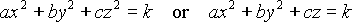The useful conics are the ellipsoid (of which the sphere is a special case), the infinite cylinder, and the infinite cone. Various hyperboloids, and paraboloids are also defined by these equations, but these have little real use unless one is designing satellite dishes (paraboloid), headlamp reflectors (also paraboloid), or power station cooling towers (hyperboloid). For more details see R&A section 6-4, especially Figure 6-18 on page 403. Figure 6-18 is included in the handout.

These are an extension of quadrics, where the power on the coordinate does not have to be 2. The general form of a superquadric centred at the origin and aligned along the coordinate axes is: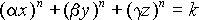Super-ellipsoids tend to be the only members of this family that are actually used, and even they are only used in very limited areas. The effect of n on a super-ellipsoid is roughly as follows: n=2 is a standard ellipsoid; n<2 is a more pointy version, the "points" being along the main axes; n>2 becomes closer to a box as n increases; n=1 is allegedly an octahedral shape; and n<1 is truly pointy along the main axes.

The interested student may like to have a quick look at Alan Barr's two papers on superquadrics. The papers can be found in the Computer Laboratory library in IEEE Transactions on Computer Graphics and Applications volume 1, number 1 (January 1981), pages 11-23, and volume 1, number 3 (July 1981), pages 41-47.

Brian Wyvill describes a use of super-ellipsoids on pages 264 and 265 of "A Computer Animation Tutorial" in Computer Graphics Techniques: Theory and Practice, Rogers and Earnshaw (editors), Springer-Verlag, 1990, ISBN 0-387-97237-4. This extract is included in the handout.

# 3A) Bezier curves

Bezier curves were covered in the Part IB Computer Graphics and Image Processing course. SMAG section 2 gives some of the mathematical details, as does R&A Section 5-8. Parts of this Section of R&A are included in the handout.

 Exercises Explain what C0-, C1-, C2-, Cn-continuity mean. Derive the constraints on control point positions which ensure that two quartic Bezier curves join with (a) C0-continuity, (b) C1-continuity, and (c) C2-continuity.

# 3B) B-splines.

B-splines are covered in some detail in SMAG section 3 and in R&A Section 5-9. Parts of this Section of R&A are included in the handout. Beware that none of the worked examples are in the handout. These may come in useful, and you will need to get hold of a real copy of R&A if you wish to work your way through them.

## Why B-splines?

B-splines have many nice properties when compared to other families of curves which could be used. They:
• minimise the order of the polynomial pieces (order k)
• maximise the continuity between pieces (continuity C(k-2))
• minimise the number of control points controlling a piece (k points)
• have positive basis functions
• have basis functions which partition unity, implying that each piece lies inside its control points' convex hull
• are invarient with respect to affine transforms

 Exercises How many control points are required for a quartic Bezier and how many for a quartic B-spline? Why are cubics the default for B-spline use? Explain the difference between Uniform, Open Uniform, and Non-Uniform knot vectors. What are the advantages of each type?

# 3C) NURBS

NURBS are covered in SMAG section 4 and in some detail in R&A Section 5-13. Parts of this Section of R&A are included in the handout.

Non-uniform rational B-splines are the curves that are currently used in any graphics application that requires curves and surfaces with more functionality than Bezier curves can offer. In addition to the features listed in Part 3B, NURBS are invarient with respect to perspective transforms.

NURBS curves incorporate -- as special cases -- uniform B-splines, non-rational B-splines, Bezier curves, lines, and conics. NURBS surfaces incorporate planes, quadrics, and tori.

 Exercises Review from IB: What are homogeneous coordinates and what are they used for in computer graphics? Explain how to use homegeneous coordinates to get rational B-splines given that you know how to produce non-rational B-splines. Convince your supervisor that you understand why NURBS includes Uniform B-splines, Non-Rational B-splines, Beziers, lines, conics, quadrics, and tori. When would you use Bezier curves and when would you use B-splines? (i.e. why have B-splines, in general, replaced Bezier curves in CAD?) [1998/7/12] Consider the design of a user interface for a NURBS drawing system. Users should have access to the full expressive power of the NURBS representation. What things should users be able to modify to give them such access and what effect does each have on the resulting shape? For each of the items (in the previous question) that the user can edit: (i) Give sensible default values; (ii) Explain how they would be constrained if a `demo' version of the software was to be limited to cubic Uniform Non-rational B-Splines. [1999/7/11] (c) Show how to construct a circle using non-uniform rational B-splines (NURBS). (d) Show how the circle definition from the previous part can be used to define a NURBS torus. [You need explain only the general principle and the location of the torus' control points.]

# 4A) Generative models

## Sweeps

These are three dimensional objects generated by sweeping a two dimensional shape along a path in 3D. Two special cases of the sweep are surfaces of revolution, where the path is a circle; and extrusions, where the path is a straight line.

Surfaces of revolution are covered in R&A section 6-2. Sweeps are covered in R&A section 6-3 and FvDFH section 12.4. Parts of Sections 6-2 and 6-3 of R&A are included in the handout.

### Extrusion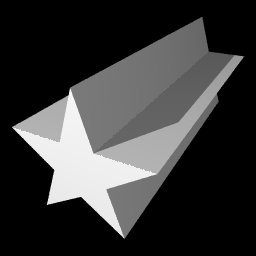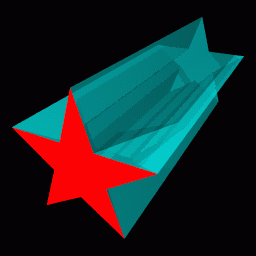On the left (above) is an example extrusion. On the right is its generating polygon (the red star), with the generated 3D object shown in semi-transparent cyan.

### Surface of revolution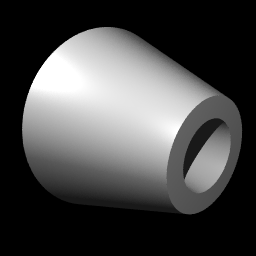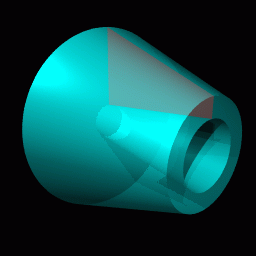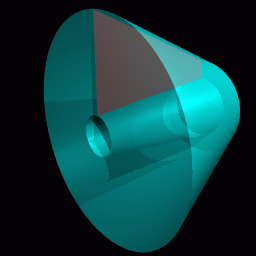On the left (above) is an example surface of revolution. On the right (above) is its generating quadrilateral (the red polygon), with the generated 3D object shown in semi-transparent cyan. Below that is another view of the same surface of revolution.

### Revolution or extrusion?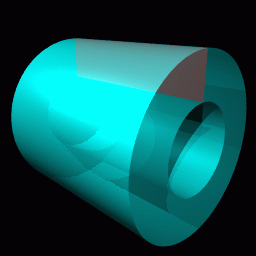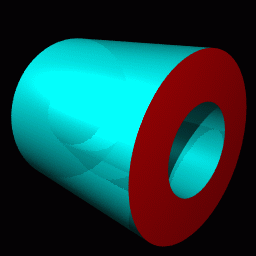Some objects can be generated in more than one way. The hollow cylinder shown above (left) could be generated as either a surface of revolution (above right) or as an extrusion (immediately right).

## General sweeps

If we push the idea of a sweep to its limit we can think of many things which could be modified to produce a three dimensional swept shape:
• Cross section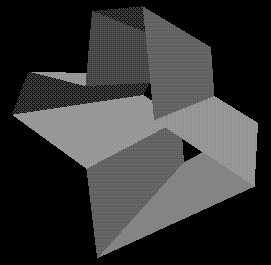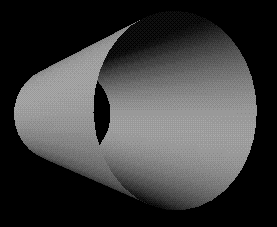Some two dimensional shape that is to be swept along the sweep path. It does not have to be circular. At right are two swept objects, one with a circular cross-section, one with a polygonal cross-section.
• Sweep path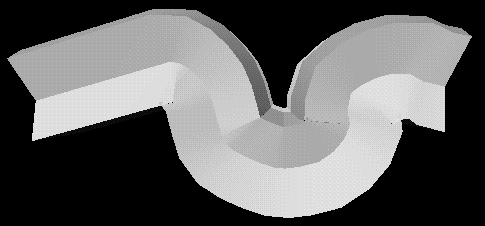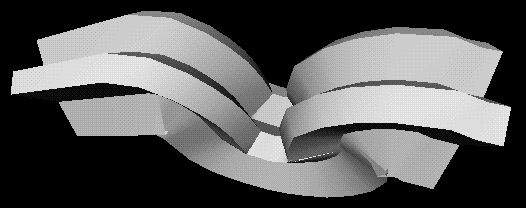The path along which the two dimensional cross section is swept to produce the three dimensional shape. It may be any curve. At right we see two views of the same swept object: a polygonal cross-section is swept along a convoluted path.
• Twist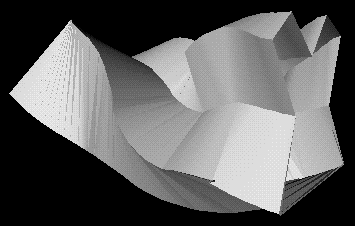How the cross section twists (rotates) as it moves along the sweep path. The default would be to have no twist at all. At right is a swept object with and without some twist.
• Scale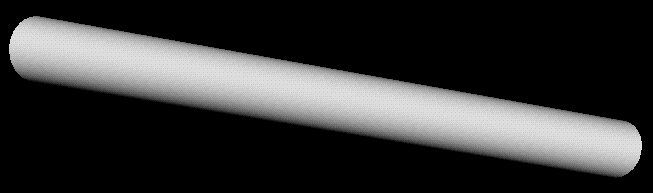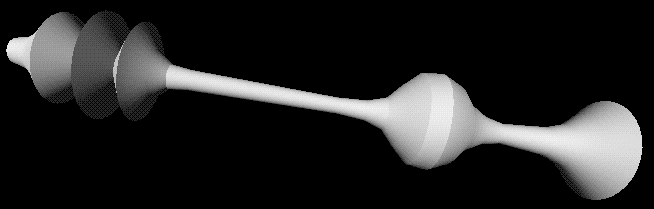How the cross section scales (changes size) as it moves along the sweep path. The default would be to have it stay the same size along the whole path. Above are a cylinder, and the same cylinder with different scales along its length.
• Normal vector direction
The normal vector of the 2D cross section will usually point along the sweep path at each point. Changing this will change the nature of the swept object. See R&A Figure 6-17 (in the handout) for an example.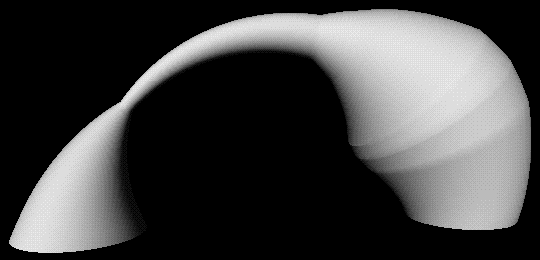At right is a swept object with a circular cross-section, semi-circular path, and varying scale.

You may be able to think of parameters, other than those in the list above, which could be modify.

 Exercises [1998/7/12] Show how the following object can be represented as a swept object.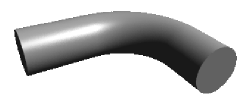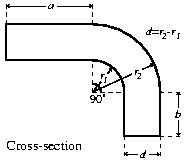Use the following different methods of specifying a geometrical model for this picture (assuming it's a three dimensional model and not a line drawing). Come as close as you can to the original for any of the methods, and describe the difficulties in using a particular method for this model.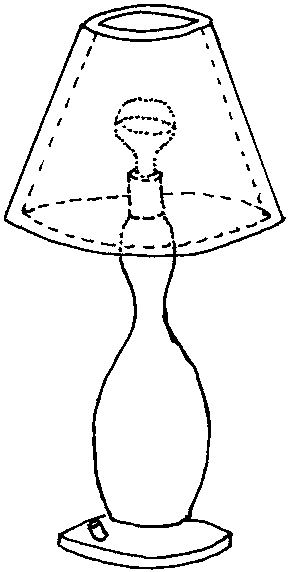Extrusions Surfaces of revolution General sweeps For each of the following categories list five real-world objects which could be represented by the primitives in the category. The ray-tracing primitives in Part 2A Extrusions Surfaces of revolution General sweeps A flume (water tunnel) at a swimming complex is modeled as a circle swept along a particular path. The designers also want to model the volume swept out by a person traveling down the flume. (We can approximate the cross-section of a person with something roughly elliptical and we'll assume the `virtual' person doesn't move legs or arms while hurtling along.) Explain which parameters in the list would be need to be modified to specify the shape of the flume and which would need to be modified to model the volume swept out by a person traveling down the flume (alternatively, specify which parameters would be held constant, in each case, for the entire length of the sweep).

# 4B) Converting swept objects to polygons

Swept objects are hard to ray trace. Imagine trying to write a ray/object intersection algorithm for a general swept object. This means that we generally need to polygonise swept objects in order to render them. For PSC we obviously must convert them to polygons.

A swept surface may be easily converted to polygons by converting the outline of the 2D cross section to a polygon, and converting the sweep path to connected set of line segments. Moving the polygon to each vertex of the set of line segments, and connecting vertices accordingly, will produced a polygon mesh which approximates the swept surface.

 Exercises [1998/7/12] Show how to convert the swept object from Part 4A Exercise 1 into polygons. What extra work would you need to do if you had to convert it into triangles?

# 4C) Constructive Solid Geometry

Constructive solid geometry (CSG) essentially consists of Boolean set operations on closed primitives in 3D space. The three CSG operations are union, intersection and difference.

CSG is covered in FvDFH sections 12.7 and 15.10.3.

The following example illustrates the three CSG operations in use on simple three dimensional primitives.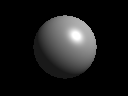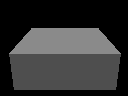The two primitives: a sphere and a box.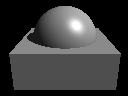The union of the two primitives.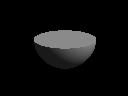The intersection of the two primitives.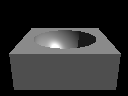The difference of the two primitives: box minus sphere.

The following example, based on FvDFH Plate III.2, shows an object for which CSG is (probably) the only sensible modelling technique. The object rendered in the right-hand image is constructed from the primitives shown in the left-hand image. It is mostly made out of cylinders, but you will recognise the extruded star from Part 4A.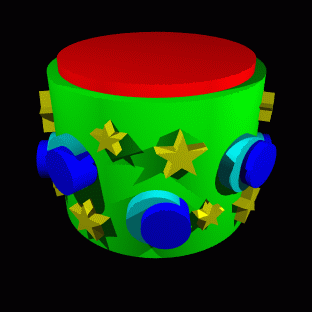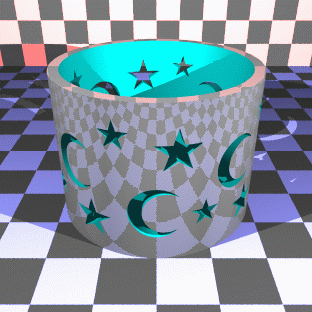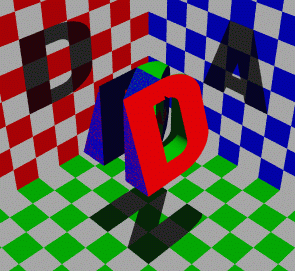At right, I tried the "Godel, Escher, Bach" treatment on my initials. Unfortunately the letters N, A, and D are not as amenable to this as the letters G, E, and B: notice that the shadow of the N has a slight curve at its top right, owing to the N's intersection with the curve on the D and the slope on the A. Various other arrangements of the three letters were tried, all of which gave more noticable artefacts than this. Each of the letters is a CSG object (the D, for example, is constructed from cylinders and boxes). The final effect is produced simply by intersecting the three letters.

 Exercises Work out how to represent a Lego technic brick as a CSG object. You may assume that you have box and cylinder primitives.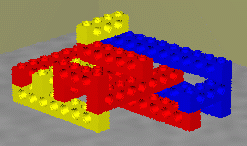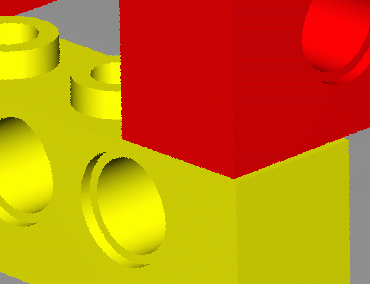[1998/7/12] Work out how to represent the following object using CSG. You may assume the following primitives: sphere, cylinder, cone, torus, box.[1999/9/4] (c) List the three ways of combining objects using constructive solid geometry (CSG). Describe how an object built using CSG can be represented using a binary tree. Given the intersection points of a ray with each primitive in the tree, explain how these points are passed up the tree by each type of combination node to produce a list of intersection points for the whole CSG object.

# 4D) Implicit surfaces, voxels and the marching cubes algorithm

## Implicit surfaces

These are covered in Brian Wyvill's article "A Computer Animation Tutorial" in Computer Graphics Techniques: Theory and Practice, Rogers and Earnshaw (editors), Springer-Verlag, 1990, ISBN 0-387-97237-4. The relevant pages of this book (258-271) are included in the handout. Note that the section on Animating SOFT Objects (pages 268-271) is for interest only, and is not examinable.

## Voxels and the marching cubes algorithm

Voxels are the three dimensional analogue of pixels. Rather than storing a colour in a voxel, you will generally store a density value. Voxels and the marching cubes algorithm are both covered in Lorenson and Cline's 1987 SIGGRAPH paper "Marching cubes: a high resolution 3D surface construction algorithm", Proc SIGGRAPH 87, pages 163-169. This paper is included in the handout. One of the reasons for including the paper is to give you a taster of what a good graphics research paper looks like. Two of the exercises relate to evaluating this paper in terms of (i) its research content and (ii) its written style..

 Exercises Give a definition of an implicit surface and give three examples of where such things might be useful. The marching cubes algorithm, as it applies to implicit surfaces, is described in the Wyvill article; while Lorenson and Cline concentrate on the algorithm as applied to voxel data. It is useful that the same algorithm works for both types of data. Explain how voxel data can be thought of a defining an implicit surface (or surfaces). Explain, conversely, how the Wyvill algorithm can be thought of as converting implicit surface data into voxel data before producing the final surface. Following Section 4 of Lorenson and Cline's paper, sketch an implementation of the two-dimensional `marching squares' algorithm -- where you generate line segments in 2D rather than triangles in 3D. An appliation of this algorithm would be the drawing of isobars on a weather map, given pressure values at a regular (2D) grid of points. Medical data is captured in slices. Each slice is a 2D image of density data. The distance between slices may be different to the distance between the pixels within a slice (for example, see Lorenson and Cline, Section 7.1, p. 167). What effect does this difference have on the voxel data? What effect does it have on the marching cubes algorithm? Consider Lorenson and Cline, Section 6. This research was done about twelve years ago. Given your knowledge of processor performance, what differences in performance would you expect to see between then and now? Lorenson and Cline is an example of a graphics research paper. Critically evaluate Lorenson and Cline. How good is this piece of research? Research papers at the SIGGRAPH conference are limited in their length. Evaluate Lorenson and Cline in terms of the following questions. What has been left out that would have been useful? What has been included that could have been left out? Where could the explanation have been better? Are any of the figures extraneous? Where would an extra figure have been helpful? List any grammatical or spelling errors (there is at least one of each).